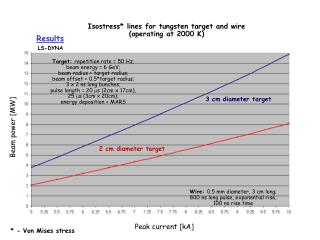DownloadDownload PresentationLS-DYNA

# LS-DYNA

Download Presentation## LS-DYNA

- - - - - - - - - - - - - - - - - - - - - - - - - - - E N D - - - - - - - - - - - - - - - - - - - - - - - - - - -
##### Presentation Transcript

1. Target: repetition rate = 50 Hz; beam energy = 6 GeV; beam radius = target radius; beam offset = 0.5*target radius; 3 x 2 ns long bunches; pulse length = 20 s (2cm x 17cm), 25 s (3cm x 20cm); energy deposition = MARS Wire: 0.5 mm diameter, 3 cm long; 800 ns long pulse, exponential rise, 100 ns rise time 3 cm diameter target 2 cm diameter target Peak current [kA] Results * - Von Mises stress Beam power [MW] Isostress* lines for tungsten target and wire (operating at 2000 K) LS-DYNA

2. Max temp = 2200 K Max temp = 1900 K Max temp = 1900 K Max temp = 2050 K Max temp = 1900 K Max temp = 2000 K + new -> (nb) = not broken 1.0 3.4(nb) 4.2(nb) 9.0(nb) 10.0(nb) [Max temp = 1900K] 0.00001 + 2.0(nb) [Max temp = 1800K] 1.6 Wire test results: Number of pulses to failure [x106] 2 cm diameter target 3 cm diameter target Peak current [kA] Beam power [MW]

3. Target: 3cm x 20cm;repetition rate = 50 Hz; beam energy = 6 GeV; 3 x 2 ns long bunches; pulse length = 25 s; beam radius = target radius; beam offset = target radius/2; energy deposition = MARS Wire: 0.5 mm diameter, 3 cm long; 800 ns long pulse, exponential rise, 100 ns rise time Temperature rise (peak value) [K] Wire Target Peak von Mises stress [MPa] Stress as a function of temperature jump in the tungsten target and wire (operating at 2000 K) LS-DYNA

4. Target: repetition rate = 50 Hz; beam energy = 6 GeV; beam radius = target radius; beam offset = 0.5*target radius; 3 x 2 ns long bunches; pulse length = 20 s (2cm x 17cm), 25 s (3cm x 20cm); energy deposition = MARS Wire: 3 cm long; 800 ns long pulse, exponential rise, 100 ns rise time. 0.75 mm diameter wire 1 mm diameter wire 1.25 mm diameter wire 2 cm diameter target 3 cm diameter target Peak current [kA] Results Beam power [MW] The current needed in a test with thicker tungsten wire in order to have the same peak stress as in a real target LS-DYNA